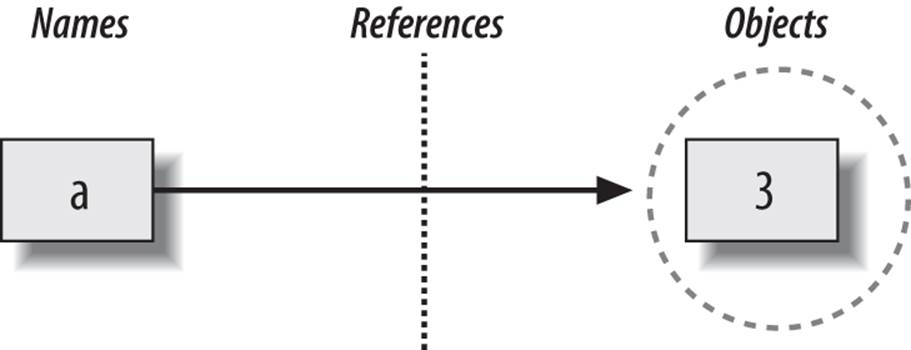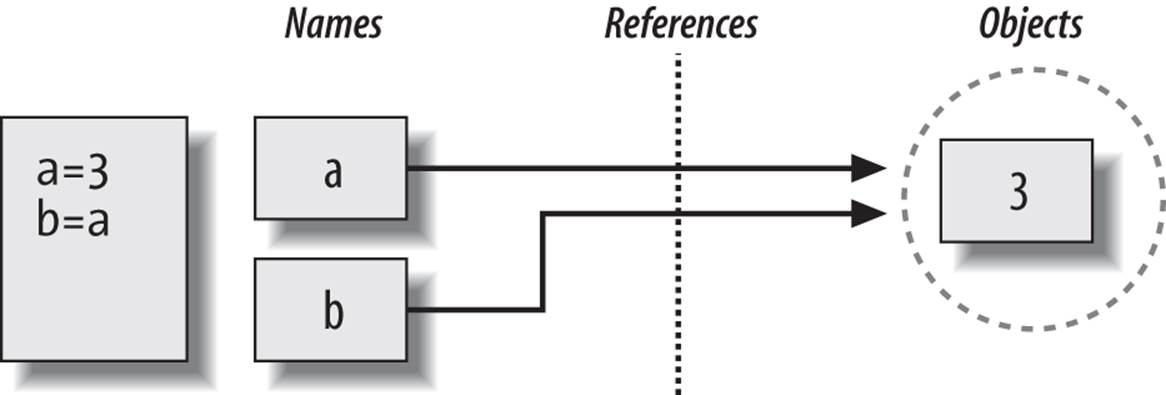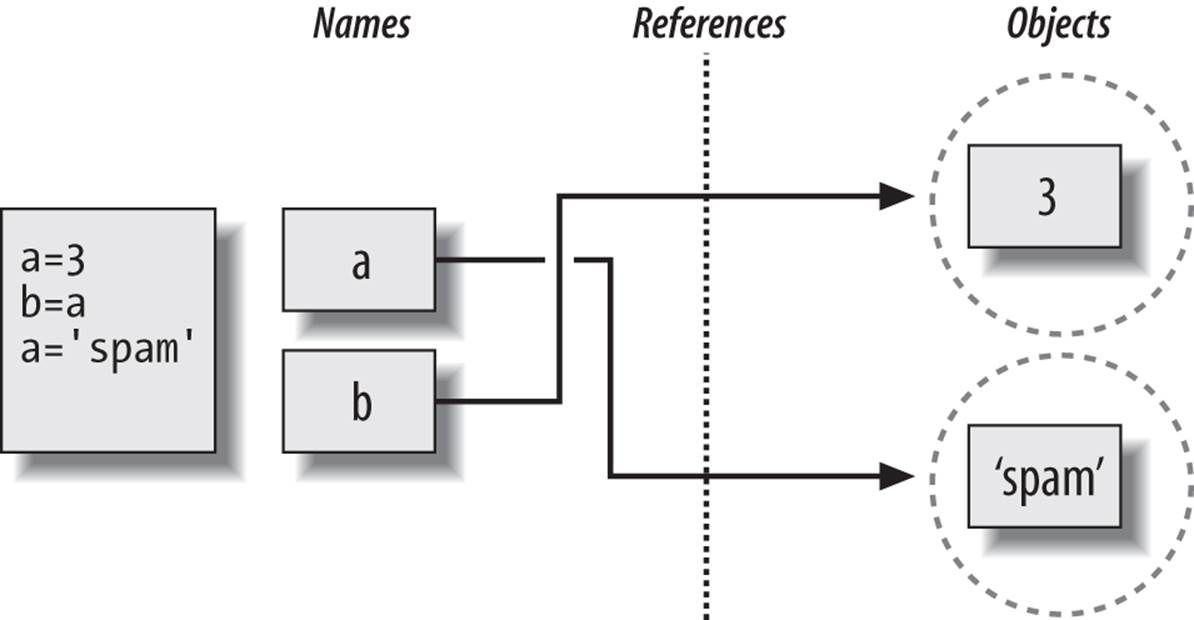# 1: Basics

This Python tutorial will cover the basics of - Jupyter Notebooks - basic types in Python - container types - control flow

# Jupyter

A Jupyter Notebook consists of cells that can be executed. There are two fundamental different types of cells, markdown or code cells. The former renders the text as markdown while the latter runs the Python interpreter.

Notebooks can be converted to Python scripts.

Be aware that notebooks are stateful. E.g. executing a cell again is like adding this line two times in a script.

Useful commands: - to run a cell and go to the next one, press Shift + Enter - to move from editing mode to navigation (move up and down in cells), press Esc - to enter editing mode from navigation, press Enter

Let’s start! Execute the lines below

:

a = 0

:

a = a + 1
print(a)

1


If we rerun the cell above, a will have changed again! If you want to rerun everythin, restart the kernel first.

Notebooks offer convenient functionality, similar to IPython: - can use !command to execute bash - has tab-completion - prints automatically return value if it is not assigned to a variable - magic commands like %timeit

We will use them later on more

## Basic types and operations

Python has several basic types - numerical (float, int, complex) - string - bool

There are several operations defined on them, as we have already seen in examples.

:

a = 1  # creates an integer

b = 3.4  # float

# several ways for strings
c = "hello"
d = 'world'
cd = "welcome to this 'world' here"  # we can now use '' inside (or vice versa)
e = """hello world"""  # which we can also wrap
e2 = """hello
world
come here!"""

g = True

:

type(a)

:

int


With type(...), we can determine the type of an object.

## strong typing

Python is strongly typed (as opposed to weakly typed). This means that the type of the variable matters and some interactions between certain types are not directly possible.

:

a = 1
b = 2

:

a + b

:

3


These are two integers. We are not surprised that this works. What about the following?

:

mix_str_int = a + "foo"

---------------------------------------------------------------------------
TypeError                                 Traceback (most recent call last)
Cell In, line 1
----> 1 mix_str_int = a + "foo"

TypeError: unsupported operand type(s) for +: 'int' and 'str'


Maybe the following works?

:

mix_str_int2 = a + "5"

---------------------------------------------------------------------------
TypeError                                 Traceback (most recent call last)
Cell In, line 1
----> 1 mix_str_int2 = a + "5"

TypeError: unsupported operand type(s) for +: 'int' and 'str'


Python is strict on the types, but we can sometimes convert from one type to another, explicitly:

:

a + int("5")

:

6


…which works because int("5") -> 5.

There are though some implicit conversions in Python, let’s look at the following:

:

f = 1.2
print(type(f))

<class 'float'>

:

int_plus_float = a + f
print(type(int_plus_float))

<class 'float'>


This is one of the few examples, where Python automatically converts the integer type to a float. The above addition actually reads as

:

int_plus_float = float(a) + f


Similar with booleans as they are in principle 1 (True) and 0 (False)

:

True + 5

:

6


For readability, it is usually better to write an explicit conversion.

## Container types

Python has several container types as also found in other languages. The most important ones are: - list (~array in other languages) - dict (~hash table in other languages)

They can contain other objects which can then be assigned and accessed via the [] operator (we will have a closer look at operators later on)

A list stores elements by indices, which are integers, while a dict stores elements by a key, which can be “any basic type” (to be precise: by their “hash”, it can be any immutable type).

Let’s look at examples!

:

# creating a list
list1 = [1, 2, 3]
print(list1)

[1, 2, 3]


We can access these element by indices, starting from 0

:

list1

:

1


We can also assign a value to an place in the list

:

list1 = 42
print(list1)

[1, 42, 3]


and it can be extended with elements

:

list1.append(-5)
print(list1)

[1, 42, 3, -5]


Choosing a value that is not contained in the list raises an error. It is verbose, read and understand it.

Being able to understand and interpret errors correctly is a key to becoming better in coding.

:

list1

---------------------------------------------------------------------------
IndexError                                Traceback (most recent call last)
Cell In, line 1
----> 1 list1

IndexError: list index out of range


We can play a similar game with dicts

:

person = {'name': "Jonas Eschle", 'age': 42, 5: True, 11: "hi"}  # we can use strings but also other elements
print(person)

{'name': 'Jonas Eschle', 'age': 42, 5: True, 11: 'hi'}

:

print(person['name'])
print(person)
print(person)

Jonas Eschle
True
hi


We can also assign a new value to a key.

:

person['age'] = '42.00001'


… or even extend it by assigning to a key that did not yet exists in the dict

:

person['alias'] = "Mayou36"
print(person)

{'name': 'Jonas Eschle', 'age': '42.00001', 5: True, 11: 'hi', 'alias': 'Mayou36'}


As we see this works. Notice, that the dict has changed, same as the list before.

Again, selecting a key that is not contained in the dict raises an error.

:

person['nationality']

---------------------------------------------------------------------------
KeyError                                  Traceback (most recent call last)
Cell In, line 1
----> 1 person['nationality']

KeyError: 'nationality'


As any object in Python, there are many useful methods on list and dict that help you accomplish things. For example, what if we want to retrieve a value from a dict only if the key is there and otherwise return a default value? We can use get:

:

hair_color = person.get('hair_color', 'unknown color')  # the second argument gets returned if key is not in dict
print(hair_color)

unknown color


## Mutability

Python has a fundamental distinction between mutable and immutable types.

Mutable means, an object can be changed Immutable means, an object can not be changed

As an example, 5 can not change; in general the basic types we looked at cannot change. We can change the value that is assigned to a variable, but the object 5 remains the same. The list and dicts we have seen above on the other hand are mutable, they have changed over the course of execution.

Every mutable object has an immutable counterpart (but not vice-versa): - list -> tuple - dict -> frozendict - set -> frozenset - etc.

:

# creating a tuple
tuple1 = (1, 3, 5)
# or from a list
tuple_from_list = tuple(list1)

:

list2 = [4, 5]
tuple2 = (3, 4)
list3 = list(tuple2)

[ ]:




While we can access the elements as we can for a list, we can neither assign nor append (or in generate mutate the object:

:

print(tuple1)  # access works!

3

:

tuple1 = 5

---------------------------------------------------------------------------
TypeError                                 Traceback (most recent call last)
Cell In, line 1
----> 1 tuple1 = 5

TypeError: 'tuple' object does not support item assignment


We will soon see the effects and needs for this…

## dynamic typing

Python is dynamically typed (as opposed to statically typed). This means that a variable, which once was an int, such as a, can be assigned a value of another type.

:

a = 1

:

a = "one"

:

a = list1


… and so on

# Assignement and variables

We’ve seen a few things up to now but have not really looked at the assignement and variables itself. Understanding Pythons variable is crucial to understand e.g. the following:

:

a = 5
b = a
print(a, b)

5 5

:

a = 3
print(a, b)

3 5


So far so good, no surprize here.

:

list1 = [1, 3]
list2 = list1
print(list1, list2)

[1, 3] [1, 3]

:

list2 = 99
print(list1, list2)

[99, 3] [99, 3]


…but that was probably unexpected! Let’s have a look at Pythons variable assignement.

## Python variable assignement

Assigning something to a variable in Python makes a name point to an actual object, so the name is only a reference. For example creating the variable a and assigning it the object 5 looks like this::

a = 3
list_a = [1, 2]:

b = a  # this assigns the reference of a to b
list_b = list_a


Both objects, b and list_b point now to the same objects in memory as a and list_a respectively. Re-assigning a variable let’s it point to a different object:

a = 'spam'
list_a = [1, 5, 2, 'world', 1]
print(a, b)
print(list_a, list_b)

spam 3
[1, 5, 2, 'world', 1] [1, 2]


Let’s make them point to the same object again:

:

b = a
list_b = list_a
print(a, b)
print(list_a, list_b)

spam spam
[1, 5, 2, 'world', 1] [1, 5, 2, 'world', 1]

:

list_a = 'hello'
print(list_a, list_b)

[1, 'hello', 2, 'world', 1] [1, 'hello', 2, 'world', 1]


Now we understand what happend: the object that both variables are pointing to simply changed. This is impossible with immutable objects (such as 3), since they are immutable.

Mutable objects usually offer the ability to create a copy.

:

list_c = list_a.copy()  # now there are two identical lists in the memory

:

list_a = 'my'
print(list_a)
print(list_b)
print(list_c)

[1, 'hello', 'my', 'world', 1]
[1, 'hello', 'my', 'world', 1]
[1, 'hello', 2, 'world', 1]


list_a and list_b, pointing to the same object that was mutated, have changed, while list_c, pointing to a different object, remained the same.

Let’s have a look at two operators: the “trivial” == and the is: we know == pretty well, it tells whether the left and the right side are the same. More specific, it tells whether both sides have/represent the same value, not whether they are in fact the same object! The operator is tells us, whether two objects are the same object (compare our assignement model above!).

:

print(list_a == list_c)  # not the same
print(list_a == list_b)  # the same

False
True

:

list_c = 'my'  # make it the same as the other lists
print(list_a == list_c)

True


But, as we learned before, they are not the same objects!

:

print(list_a is list_c)  # nope!
print(list_a is list_b)  # yes!

False
True


Normally, we are only interested to compare the values, using ==.

## Sugar: comprehensions

List comprehensions

:

N = 10

list_of_squares = [i**2 for i in range(N)]
sum_of_squares = sum(list_of_squares)

print('Sum of squares for', N, 'is', sum_of_squares)

Sum of squares for 10 is 285

:

# [obj_to_return if ... else ... for i in ... for j in ... if ...]
[i for i in range(10) if i % 2]

:

[1, 3, 5, 7, 9]

:

a

:

'spam'


Dictionary comprehensions

:

squares = {i: i**2 for i in range(10)}
print(squares)

{0: 0, 1: 1, 2: 4, 3: 9, 4: 16, 5: 25, 6: 36, 7: 49, 8: 64, 9: 81}

:

N = 5
print('The square of', N, 'is', squares[N])

The square of 5 is 25


## Sugar: using Markdown

Use LaTeX:

$$A = \frac{1}{B+C}$$

Show lists:

• A wonderful

• List

• This is

Show code with syntax highlighting:

Python: (but in a sad grey world)

print('Hello world')


Python:

print('Hello world')


C++:

#include <iostream>

std::cout << "Hello world" << std::endl;


Bash:

echo "Hello world"


f-strings

:

pt_cut = 1789.234567890987654
eta_low = 2
eta_high = 5

cut_string = f'(PT > {pt_cut:.2f}) & ({eta_low} < ETA < {eta_high})'
print(cut_string)

(PT > 1789.23) & (2 < ETA < 5)